IntroductionIntroduction# Volume and Surface Area of Spheres

INTRO
Volume tells us the amount of space inside a given 3D shape. Surface area tells us the area of the surface of a 3D shape.
Think of it in terms of an apple. The volume is the total amount of apple, and the surface area is the amount of skin on the apple.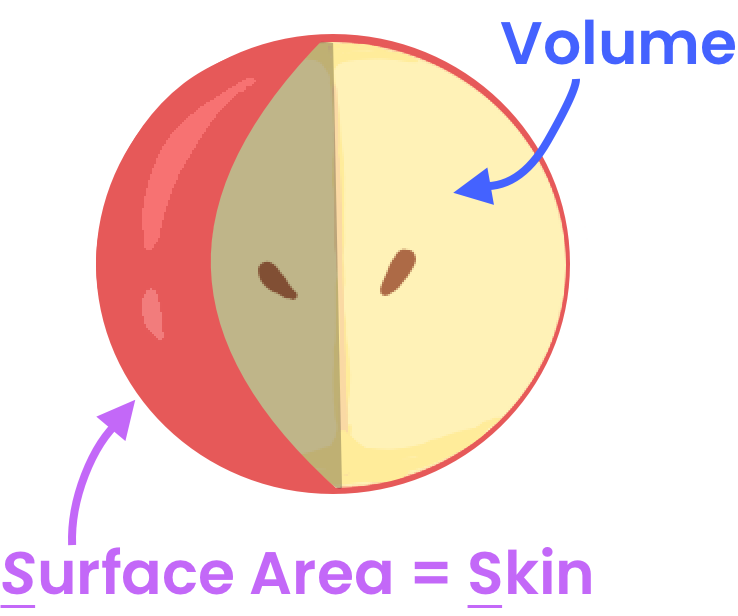CALCULATOR

## Volume and Surface Area of Spheres Calculator

### Step 1. Determine if you’re solving for volume (V) or surface area (SA).KEY STEPS

## How to Find the Volume and Surface Area of a Sphere

### Step 1. Determine if you’re solving for volume (V) or surface area (SA).

What are you trying to solve for?
We're looking for the volume, or the total amount of space inside the sphere, which is like the total amount of apple.

### Step 2. Find the radius of the sphere.

The radius is the distance from the center of the sphere to the surface.

### Step 3. Calculate the volume with the radius.

LESSON
Volume of Spheres

## Volume of Spheres

Finding the volume of a sphere is like finding the total amount of fruit inside the apple, and the only thing we need to know in order to solve for the volume of a sphere is its radius.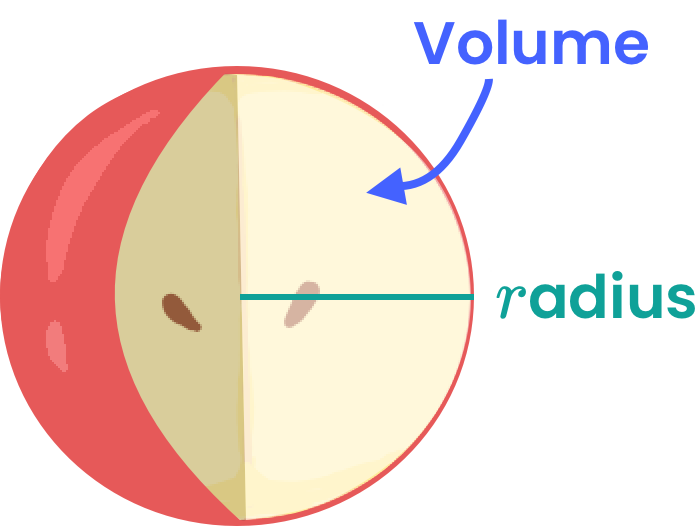Let’s put this formula to use by doing a couple example problems where we solve for the volume of a sphere!
PRACTICE
Volume of Spheres

## Practice: Volume of a Sphere

Question 1 of 3: What is the volume of a sphere with ?

### Step 1. Determine what you are solving for.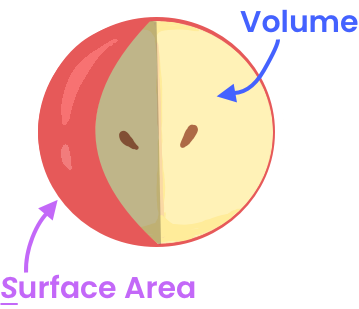Finding the volume of a sphere is like finding the total amount of an apple.

LESSON
Surface Area of Spheres

## Surface Area of Spheres

Finding the surface area of a sphere is like finding the total amount of skin on the apple, and the only thing we need to know in order to solve for the surface area of a sphere is its radius.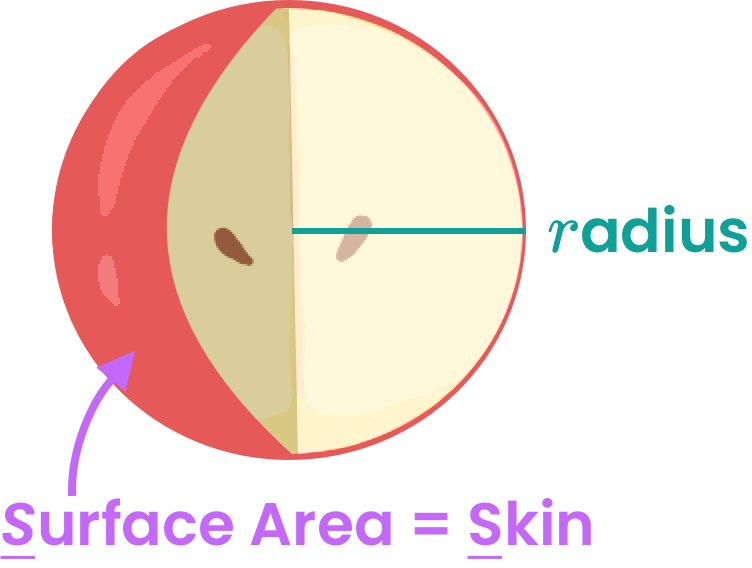### Help Me Remember This 🙏🏿🙏🏻🙏🏽

You can imagine that the sphere is being wrapped around by a cylinder: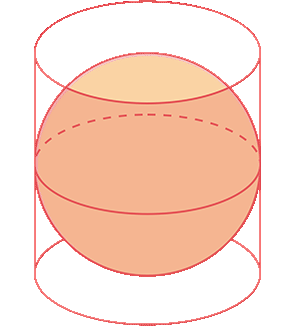The surface area of the sphere is equal to the area of the rectangle we use to wrap the sphere: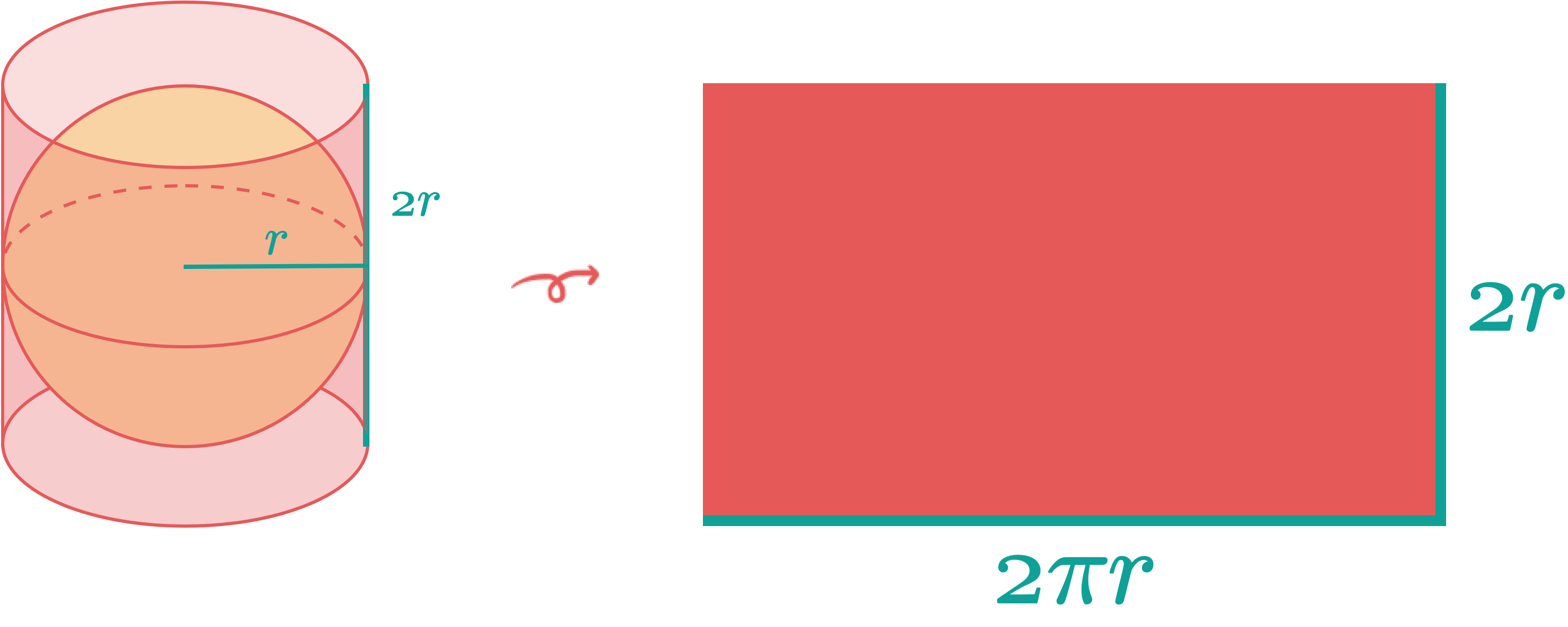Let’s put this formula to use by doing a couple example problems where we solve for the surface of a sphere!
PRACTICE
Surface Area of Spheres

## Practice: Surface Area of a Sphere

Question 1 of 3: What is the surface area of a sphere with ?

### Step 1. Determine what you are solving for.Finding the surface area of a sphere is like finding the total amount of an apple.

CONCLUSION
Dang, look at you go! Thanks for checking out this lesson ☺️🙏. Where to next?Leave Feedback